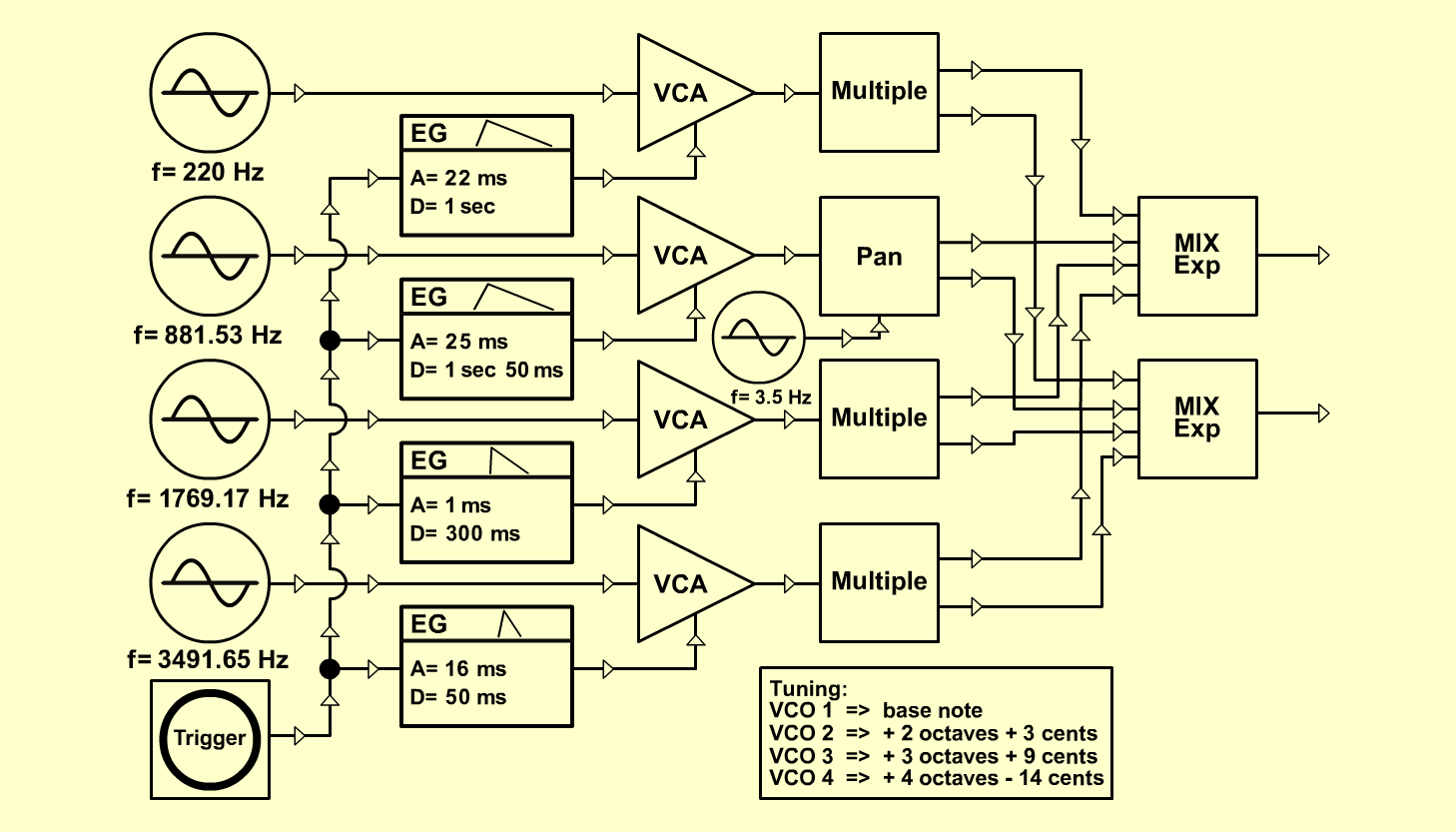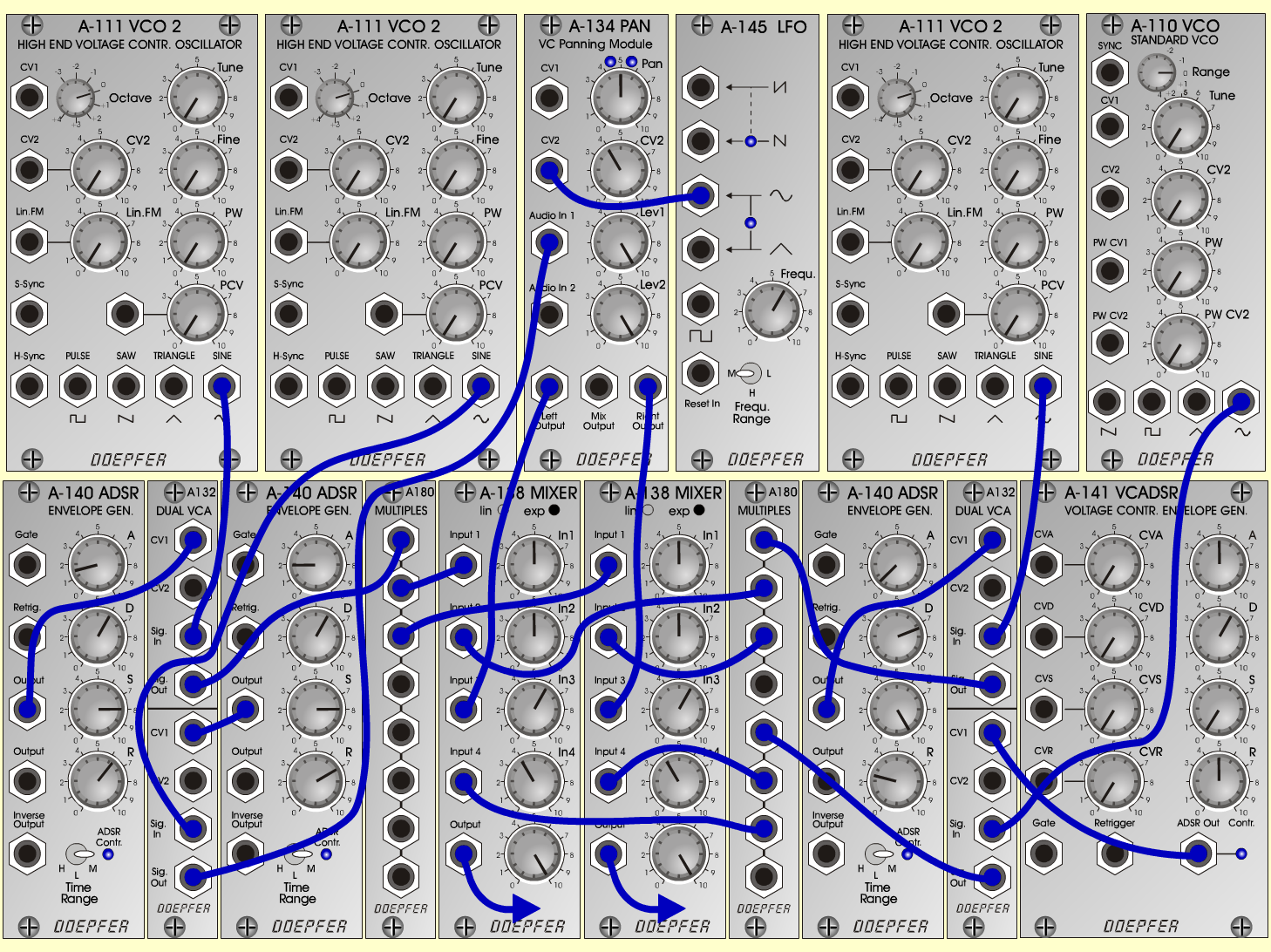MarimbaInstrumentsD o e p f e r A - 100 Connections: Settings: A-111/1 (Sine) <=> A-132/1upper (Sig. In) A-111/2 (Sine) <=> A-132/1lower (Sig. In) A-111/3 (Sine) <=> A-132/2upper (Sig. In) A-110 (Sine) <=> A-132/2lower (Sig. In) A-140/1 (Output) <=> A-132/1upper (CV 1) A-140/2 (Output) <=> A-132/1lower (CV 1) A-140/3 (Output) <=> A-132/2upper (CV 1) A-141 (Output) <=> A-132/2lower (CV 1) A-132/1upper (Sig Out) <=> A-180/1 A-132/1lower (Sig Out) <=> A-134 (Audio In 1) A-132/2upper (Sig Out) <=> A-180/2 A-132/2lower (Sig Out) <=> A-180/3 A-180/1 <=> A-138/1exp (Input 1) A-180/1 <=> A-138/2exp (Input 1) A-180/2 <=> A-138/1exp (Input 2) A-180/2 <=> A-138/2exp (Input 2) A-180/3 <=> A-138/1exp (Input 4) A-180/3 <=> A-138/2exp (Input 4) A-134 (Left Out) <=> A-138/1exp (Input 3) A-134 (Right Out) <=> A-138/2exp (Input 3) A-145 (Sine) <=> A-134 (CV 2) A-138/1exp (Output) <=> Amplifier (Left) A-138/2exp (Output) <=> Amplifier (Right) A-176 (CV 1) <=> A-110 (VC 1) A-176 (CV 2+3) <=> A-111/2+3 (CV1) Trigger: A-146 (Pulse) <=> A-185 (Gate In) A-185 (Gate => Bus) <=> A-140/1-3 (Bus => Gate) A-185 (Gate) <=> A-141 (Gate) A-140/1 (A = 1.5, D = 6, S = 8, R = 6.3, Range = M) A-140/2 (A = 2, D = 6, S = 8, R = 7, Range = M) A-140/3 (A = 0.5, D = 7.3, S = 10, R = 2.5, Range = M) A-141 (A = 5, D = 6, S = 0, R = 5) A-138/1exp (In 1 = 5, In 2 = 5, In 3 = 6, In 4 = 4, Out = 10) A-138/2exp (In 1 = 5, In 2 = 5, In 3 = 6, In 4 = 4, Out = 10) A-145 (Frq = 6, Range = M) Tuning:A-111/1 => Base NoteA-111/2 => + 2 octaves + 3 centsA-111/3 => + 3 octaves + 9 centsA-110 => + 4 octaves - 14 cents Josef MuellerSound samples Marimba-Piece Marimba-Piece (with chorus and reverb) Marimba (65 Hz) Marimba (139 Hz) Marimba (261 Hz) Marimba (523 Hz)
br>